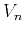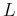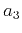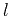Nonhyperbolic reflection moveout of-waves: An overview and comparison of reasonsNext: Curved reflector beneath homogeneous Up: CURVILINEAR REFLECTOR Previous: CURVILINEAR REFLECTOR

## Curved reflector beneath isotropic medium

If the reflector has the shape of a dipping plane beneath a homogeneous isotropic medium, the reflection moveout in the dip direction is a hyperbola (Levin, 1971)(53)

Here(54)(55)is the length of the zero-offset ray, andis the reflector dip. Formula (53) is inaccurate if the reflector is both dipping and curved. The Taylor series expansion for moveout in this case has the form of equation (22), with coefficients (Fomel, 1994)(56)(57)

where(58)is the reflector curvature [defined by equation (61)] at the reflection point of the zero-offset ray, andis the third-order curvature [equation (62)]. If the reflector has an explicit representation, then the parameters in equations (56) and (57) are(59)(60)(61)(62)

Keeping only three terms in the Taylor series leads to the approximation(63)

where we included the denominator in the third term to ensure that the traveltime behavior at large offsets satisfies the obvious limit(64)

As indicated by equation (61), the sign of the curvatureis positive if the reflector is locally convex (i.e., an anticline-type). The sign ofis negative for concave, syncline-type reflectors. Therefore, the coefficientexpressed by equation (58) and, likewise, the nonhyperbolic term in (63) can take both positive and negative values. This means that only for concave reflectors in homogeneous media do nonhyperbolic moveouts resemble those in VTI and vertically heterogeneous media. Convex surfaces produce nonhyperbolic moveout with the opposite sign. Clearly, equation (63) is not accurate for strong negative curvatures, which cause focusing of the reflected rays and triplications of the reflection traveltimes.

In order to evaluate the accuracy of approximation (63), we can compare it with the exact expression for a point diffractor, which is formally a convex reflector with an infinite curvature. The exact expression for normal moveout in the present notation is(65)

whereis the depth of the diffractor, andis the angle from vertical of the zero-offset ray. Figure 4 shows the relative error of approximation (63) as a function of the ray angle for offsettwice the diffractor depth. The maximum error of about 1% occurs at. We can expect equation (63) to be even more accurate for reflectors with smaller curvatures.nmoerr
Figure 4.
Relative errorof the nonhyperbolic moveout approximation (63) for a point diffractor. The error corresponds to offsettwice the diffractor depthand is plotted against the angle from verticalof the zero-offset ray.Nonhyperbolic reflection moveout of-waves: An overview and comparison of reasonsNext: Curved reflector beneath homogeneous Up: CURVILINEAR REFLECTOR Previous: CURVILINEAR REFLECTOR

2014-01-27